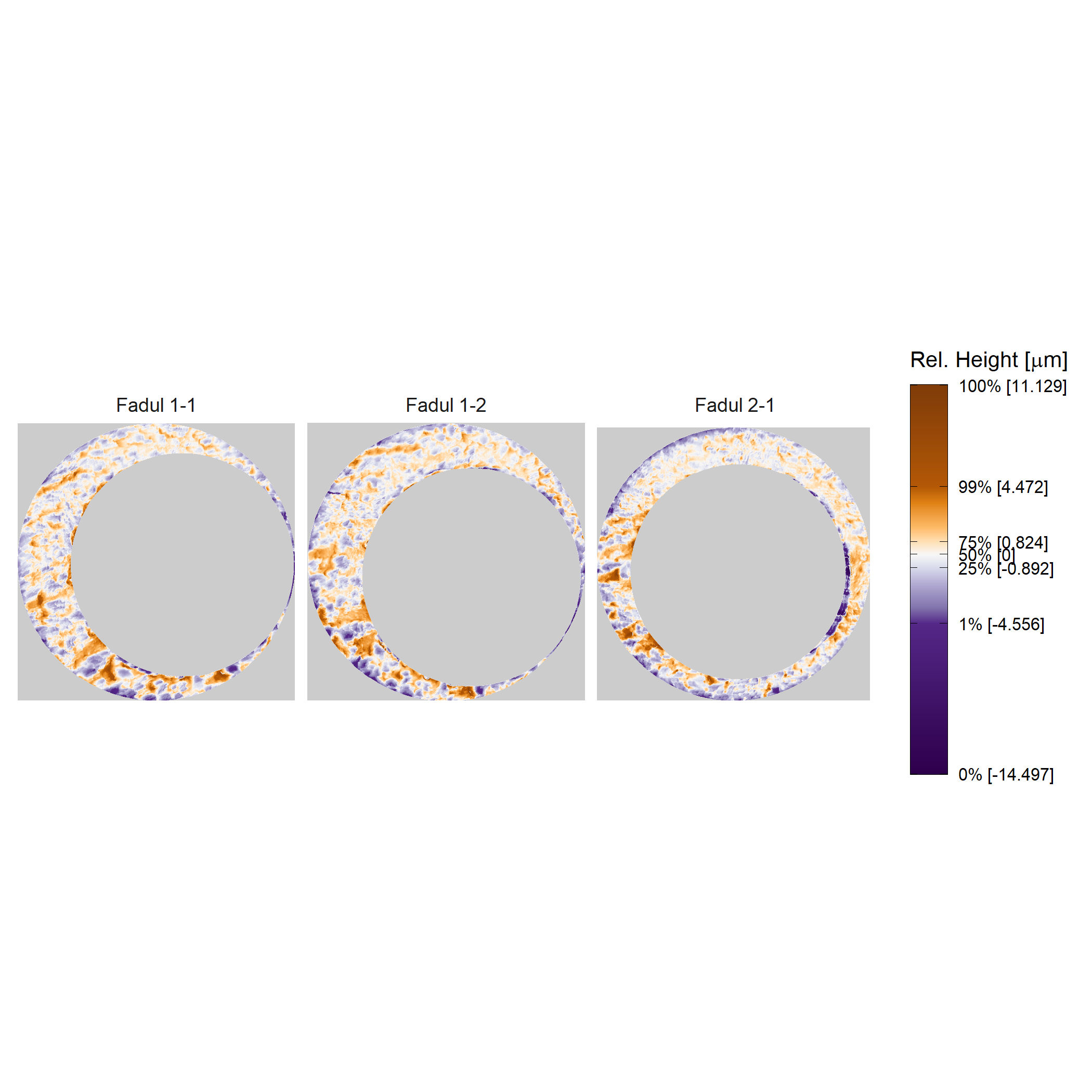# Set-up

This vignette will describe the decision rules used in the original method of Song (2013) and the High CMC method of Tong et al. (2015). For illustrative purposes, we will consider a comparison between a known match and known non-match pair of cartridge cases from the stuy performed by Fadul et al. (2011). The raw cartridge case scans can be downloaded from the NIST Ballistics Toolmark Research Database. The scans were preprocessed using functions available in the cmcR package and are not discussed here. Refer to the fadul_examples.R script available on the cmcR GitHub page for how these scans were preprocessed. We will also not discuss how similarity features are extracted from two processed scans. Refer to the documentation of the comparison_allTogether function on the cmcR website for information regarding this procedure.

library(cmcR)
library(dplyr)
library(ggplot2)
library(purrr)
library(tidyr)
library(gridExtra)

We will consider comparisons between three cartridge case scans. Fadul 1-1 and Fadul 1-2 are known matches (i.e., were fired from the same firearm) while Fadul 2-1 is a non-match. The comparisons considered are Fadul 1-1 vs. Fadul 1-2 and Fadul 1-1 vs. Fadul 2-1.

data("fadul1.1_processed")
data("fadul1.2_processed")
#Download a non-matching cartridge case to Fadul 1-1 and Fadul 1-2

preProcess_crop(region = "exterior",
preProcess_crop(region = "interior",
preProcess_removeTrend(statistic = "quantile",
tau = .5,
method = "fn") %>%
preProcess_gaussFilter() %>%
x3ptools::sample_x3p()

The three processed cartridge cases are shown below.The cell-based comparison procedure implemented in the comparison_allTogether function returns a data frame/tibble of similarity features between two cartridge case scans. For each cell in the “reference” scan (Fadul 1-1 in this example), the similarity features include

• Estimated horizontal and vertical translations, (x,y), required to align the reference cell in the target scan
• Cross-correlation between the reference cell and its associated target region after aligning by the estimated (x,y) values
• Rotation performed on the target scan that resulted in the (x,y,CCF) feature set

The fundamental assumption underlying all CMC decision rules is that truly matching cartridge case pairs should have similarity features that are consistent across the cell/region pairs. In particular, a plurality of cell/region pairs should “vote” for similar (x, y, theta) alignment values. In contrast, the cell/region pairs of a truly non-matching cartridge cases should have seemingly random (x, y, theta) votes. The two decision rules implemented in the cmcR package can be understood as two different systems by which cells vote for (x, y, theta) values that they “believe” to be the true alignment values for the overall cartridge case scans.

# Original method of Song (2013)

An actual implementation of the original method of Song (2013) is described in Song et al. (2014). The decision rule Song et al. (2014) describe using is based on

a virtual reference with three reference registration parameters $$\theta_{\text{ref}}$$, $$x_{\text{ref}}$$ and $$y_{\text{ref}}$$ generated by the median values of the collective $$\theta$$, and $$x$$-, $$y$$-translation values of all cell pairs.

That is, a consensus is determined by finding the median registration phase values across the cell/region pairs for a particular cartridge case pair comparison. Then, the distances between the consensus registration values and the cell comparison values are assessed to determine whether they are within a specified distance of the consensus. This consensus assessment introduces threshold parameters $$T_{x}, T_{y}, T_\theta, T_{\text{CCF}}$$.

Let $$x_i, y_i, \theta_i$$ denote the translation and rotation parameters which produce the highest CCF for the alignment of cell/region pair $$i$$. Also let $$x_{\text{ref}}, y_{\text{ref}}, \theta_{\text{ref}}$$ be the median over alignment values for a particular cartridge case comparison (these are the “virtual reference” values). A cell/region pair $$i$$ is declared a match if all of the following conditions hold:

• $$|x_i - x_{\text{ref}}| \leq T_{x}$$
• $$|y_i - y_{\text{ref}}| \leq T_{y}$$
• $$|\theta_i - \theta_{\text{ref}}| \leq T_{\theta}$$
• CCF$$_{\max,i} \geq T_{\text{CCF}}$$.

With respect to the voting system analogy, we might interpret this decision rule as a single-choice voting system similar to the system used in U.S. presidential elections. That is, every cell is allowed to submit one vote corresponding to the registration phase with the highest CCF$$_{\max}$$ value. Some of these votes are discarded if the associated CCF$$_{\max}$$ are below the $$T_{\text{CCF}}$$ threshold. A consensus is determined by counting the number of votes that are close to the reference values $$x_{\text{ref}}, y_{\text{ref}}, \theta_{\text{ref}}$$ (which is dyadically defined based on the $$T_x,T_y,T_{\theta}$$ thresholds).

The plot below shows the values of $$x_i, y_i, \theta_i$$, and $$CCF_{\max,i}$$ for each cell/region pair between Fadul 1-1 and Fadul 1-2 as well as Fadul 1-1 and Fadul 2-1. These values are shown as blue/red bars. The purple bands indicate the range of acceptable values within $$T_{x} = 20 T_{y}, T_{\theta} = 6$$ within $$x_{\text{ref}}, y_{\text{ref}}, \theta_{\text{ref}}$$ and above $$T_{\text{CCF}} = .5$$ to be declared “congruent.” As we might expect, a larger proportion of $$x_i, y_i, \theta_i$$, and $$CCF_{\max,i}$$ values are within these acceptable ranges for the comparison between Fadul 1-1 and Fadul 1-2 than the comparison between Fadul 1-1 and Fadul 2-1. This indicates that there is a clearer “consensus” about the true alignment values for the matching cartridge case pair than the non-matching.